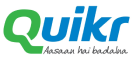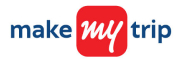New update is available. Click here to update.
Topics

Moderate0/80
Average time to solve is 10m+24 more companies

## Problem statement

You have been given two singly Linked Lists, where each of them represents a positive number without any leading zeros.

Example:
``````If the first linked list is 1 -> 2 -> 3 -> 4 -> 5 -> NULL and the second linked list is 4 -> 5 -> NULL.

The two numbers represented by these two lists are 12345 and 45, respectively. So, adding these two numbers gives 12390.

So, the linked list representation of this number is 1 -> 2 -> 3 -> 9 -> 0 -> NULL.
``````
Detailed explanation ( Input/output format, Notes, Images )
Constraints:
``````1 <= T <= 100
1 <= L <= 5000
0 <= data <= 9 and data != -1

Where 'L' is the number of nodes in either of the two Linked List and 'data' is the element value in a node of the linked list.

Time limit: 1 sec
``````
Sample Input 1 :
``````2
1 1 -1
9 9 9 -1
2 4 -1
5 3 -1
``````
Sample Output 1:
``````1 0 1 0 -1
7 7 -1
``````
Explanation for Sample Output 1:
``````In test case 1, we are adding 11 and 999 to get 1010.

In test case 2, we are adding 24 and 53 to get 77.
``````
Sample Input 2:
``````2
3 8 1 2 9 -1
9 8 2 9 -1
1 9 0 -1
8 1 0 -1
``````
Sample Output 2:
``````4 7 9 5 8 -1
1 0 0 0 -1
``````
Explanation for Sample Output 2:
``````In test case 1, we are adding 38129 and 9829 to get 47958.

In test case 2, we are adding 190 and 810 to get 1000.
``````Console